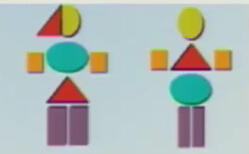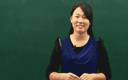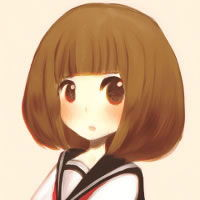### 四年级英语上册Module 4 Unit 11《Shapes》（Period 1）

• 同步课程
• 本节重点
• 主讲老师

《Shapes》（Period 1）
Let's count
How many shapes are there?
There are eight.

Look and learn
circle      square
triangle    rectangle
star

Listen and say
How many squares are there?
There are two squares.
How many circles are there?
There are six circles.
How many stars are there?
There are one star.
How many rectangles and triangles are there?
There are nine rectangle and one triangle.

Look and say
How many ____s are there?
There is/are ______.
□ There are two squares.
○ There are six circles.
☆ There are one star.
▆和△ There are nine rectangle and one triangle.

Listen and say
Miss Fang:Look at the picture.How many squares are there?
Joe:There are two squares.
Miss Fang:How many circles are there?
Peter:There are six circles.
Miss Fang:How many stars are there?
Kitty:There is one star.
Miss Fang:How many rectangles and triangles are there?
Alice:There are nice rectangles and one triangle.

Let's count
How many ________s are there?
There is/are ________.
How many ________s are there?
There is/are ________.

Quick response
How many ________s are there?
There is/are ________.
star 5      square 4       circle 3
How many ______s are there?
There is /are _________.

Think and writeHow many ______are there? _________ circles.
________ are there? _______ there _______.
________________________? There are _______.
________________________? _________________.

Listen and circle
1.There are five bookd/seven books/ten pwncils on the desk.
2.There are ten/fifteen/twenty pupiils in the copmuter room.
3.The tige is happy/not well/hungry today.
4.Ben's robot has five/ten/twenty squares,five stars/triangles/circles and six stars/triangles/circles.

Listen and judge
1.Look at my robot.It has a head.It is a circle.It has two eyes.They are squares.It has amouth.It is a star.Its body and its feet are squares.
2.Look at this robot.Its head is a square,It has theee eyes.They are squares.Itsmouth is a square too.But its feet are triangles.

Listen and circle
1.Joe:What's on the desk?
Peter:There are some books.
Joe:How many books are there?
Peter:There are seven books.
2.Alice:Whereare the pupils?
Kitty:They're in the computer room.
Alice:How many pupils are there?
Kitty:THere are twenty pupils.
3.Miss Fang:How many tiger are there in the zoo?
Joe:I see one tiger.
Miss Fang:How is the tiger?
Joe:It's not well today.It's tired,I think.
4.Ben:Look at my robot.
Kitty:Cool!A robot of shapes!How many squares are there?
Ben:One,two,three...There are ten squares.
Kitty:How many circles and triangles are there?
Ben:Let me see.There are five circles and six triangles.[广东省深圳市] 好 好 好好。136****5802

2016-11-23 12:00:43

©2016 同桌100 All Rights Reserved